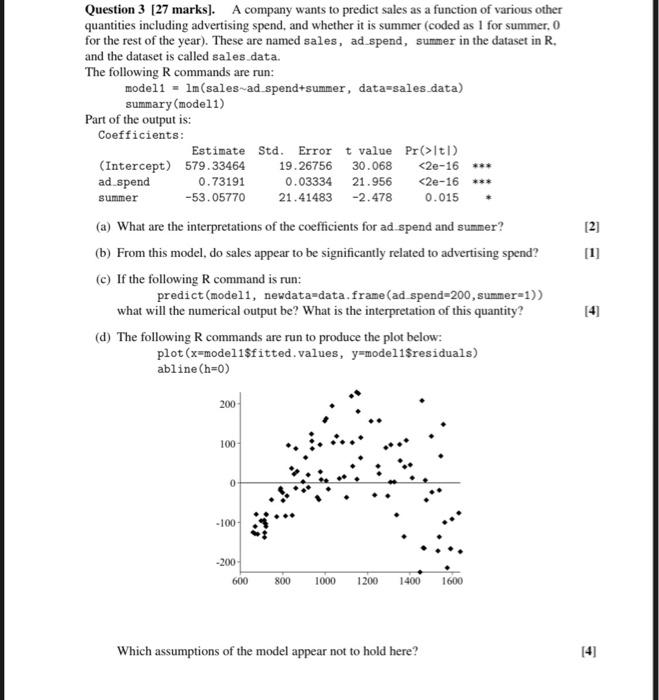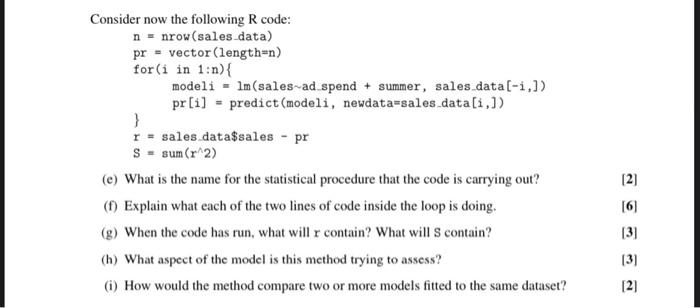Home / Expert Answers / Statistics and Probability / solve-all-parts-since-this-is-only-1-question-question-3-27-marks-a-company-wants-to-predict-sal-pa161

# (Solved): solve all parts since this is only 1 question. Question 3 [27 marks]. A company wants to predict sal ...

solve all parts since this is only 1 question.Question 3 [27 marks]. A company wants to predict sales as a function of various other quantities including advertising spend, and whether it is summer (coded as 1 for summer. 0 for the rest of the year). These are named sales, ad spend, summer in the dataset in , and the dataset is called sales_data. The following commands are run: model1 = (sales ad spend+summer, data=sales_data) summary (model1) Part of the output is: Coefficients : (a) What are the interpretations of the coefficients for ad spend and summer? (b) From this model, do sales appear to be significantly related to advertising spend? (c) If the following command is run: predict (model1, newdata=data, frame (ad spend , summer=1)) what will the numerical output be? What is the interpretation of this quantity? (d) The following commands are run to produce the plot below: plot ( modeli\$fitted, values, model1\$residuals) abline Which assumptions of the model appear not to hold here? Consider now the following R code: nrow (sales_data) vector (length=n) for in modeli sales ad_spend + summer, sales_data , \} predict (modeli, newdata=sales_data , data sales - pr (e) What is the name for the statistical procedure that the code is carrying out? (f) Explain what each of the two lines of code inside the loop is doing. (g) When the code has run, what will contain? What will S contain?  (h) What aspect of the model is this method trying to assess?  (i) How would the method compare two or more models fitted to the same dataset? 

We have an Answer from Expert